## What is Average?

Practice Unlimited Questions

#### Formula to Calculate Average:

Step 1: Find the sum or total of all items.
Step 2: Divide the total by the number of items.
Average  =
 total of all items number of items

#### 1.  Joe uses building blocks to make 3 towers of different heights as shown below. Find the average height of each tower.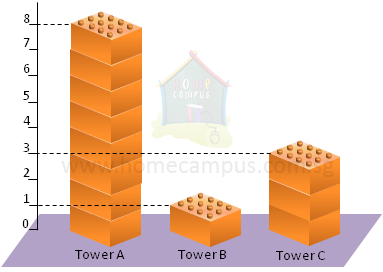• Tower A has 8 blocks
• Tower B has 1 block
• Tower C has 3 blocks
8  +  1  +  3  =  12
Joe has 12 blocks in all.

He distributes them unequally to get 3 towers of different heights (as in the figure above).

However, if he redistributes the 12 blocks equally so that each tower has the same height, then he would have found the average height of the towers (as in the figure below).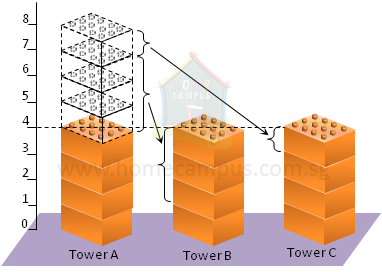Now,
• Tower A has 4 blocks
• Tower B has 4 block
• Tower C has 4 blocks

So, the average of 8, 1 and 3 is 4.

We say,
On the average, each tower has 4 blocks.

Or,
The average number of blocks per tower is 4.
Redistributing the blocks does not change the total number of blocks.
4  +  4  +  4  =  12

#### 2. The table below shows the books that Louis borrowed from the library. What is the average number of pages that each book has?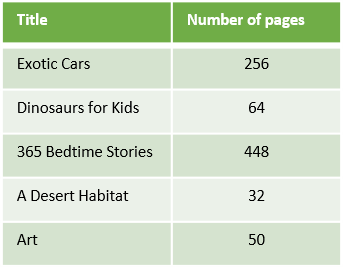Total number of pages  =  256  +  64  +  448  +  32  +  50  =  850
Number of books  =  5
Average number of pages per book  =  850  ÷  5  =  170

Each book has an average of 170 pages.

#### 3. The average age of 3 girls is 11 years. The average age of 2 of the girls is 12 years. What is the age of the third girl?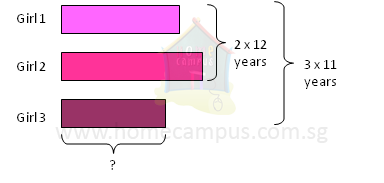3  ×  11 years  =  33 years
The total age of the 3 girls is 33 years.

2  ×  12 years  =  24 years
The total age of 2 of the girls is 24 years.

33 years  −  24 years  =  9 years
The age of the third girl is 9 years.

#### 4. A team of 5 friends gets an average pocket money of \$15 per week. A sixth friend who gets \$21 as pocket money per week joins their team. What is the average pocket money of the team now?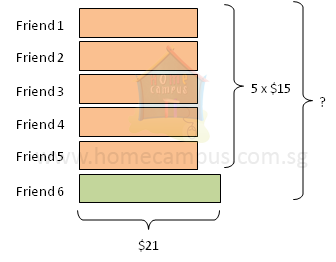5  ×  \$15  =  \$75
The total pocket money of 5 friends is \$75.

\$75  +  \$21  =  \$96
The total pocket money of 6 friends is \$96.

\$96  ÷  6  =  \$16
The average pocket money of the team now is \$16.

#### 5. Amy, Dennis and Ruby have an average salary of \$2650 per month. Amy earns twice as much as Dennis and Ruby earns \$260 more than Amy each month. What is Ruby's monthly salary?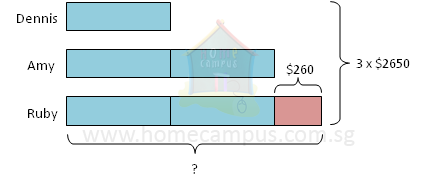3  ×  \$2650  =  \$7950
The total monthly salary of Amy, Dennis and Ruby is \$7950.

5 units  ->  \$7950  −  \$260  =  \$7690
1 unit   ->  \$7690  ÷  5  =  \$1538

2  ×  \$1538  +  \$260  =  \$3336
Ruby's monthly salary is \$3336.

#### 6. The following graph shows the number of children in 205 families in a township in the year 2009. a)  What was the total number of children in the township in 2009? b)  What was the average number of children per family in 2009? Round off your answer to 1 decimal place. c)  In the year 2010, the number of children in the township increased by 56 while the total number of families remained unchanged. What was the average number of children per family in the township in 2010? Round off your answer to 1 decimal place.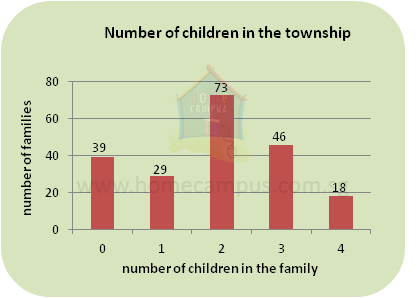a)  (39  ×  0)  +  (29  ×  1)  +  (73  ×  2)  +  (46  ×  3)  +  (18  ×  4)  =  385
The total number of children in the township in 2009 was 385.
b)  385  ÷  205  =  1.87  ≈  1.9
The average number of children per family in the township in 2009 was 1.9.
c)  385  +  50  =  435
Total number of children in the township in the year 2010 was 435.

435  ÷  205  =  2.12  ≈  2.1
The average number of children per family in the township in 2010 was 2.1.